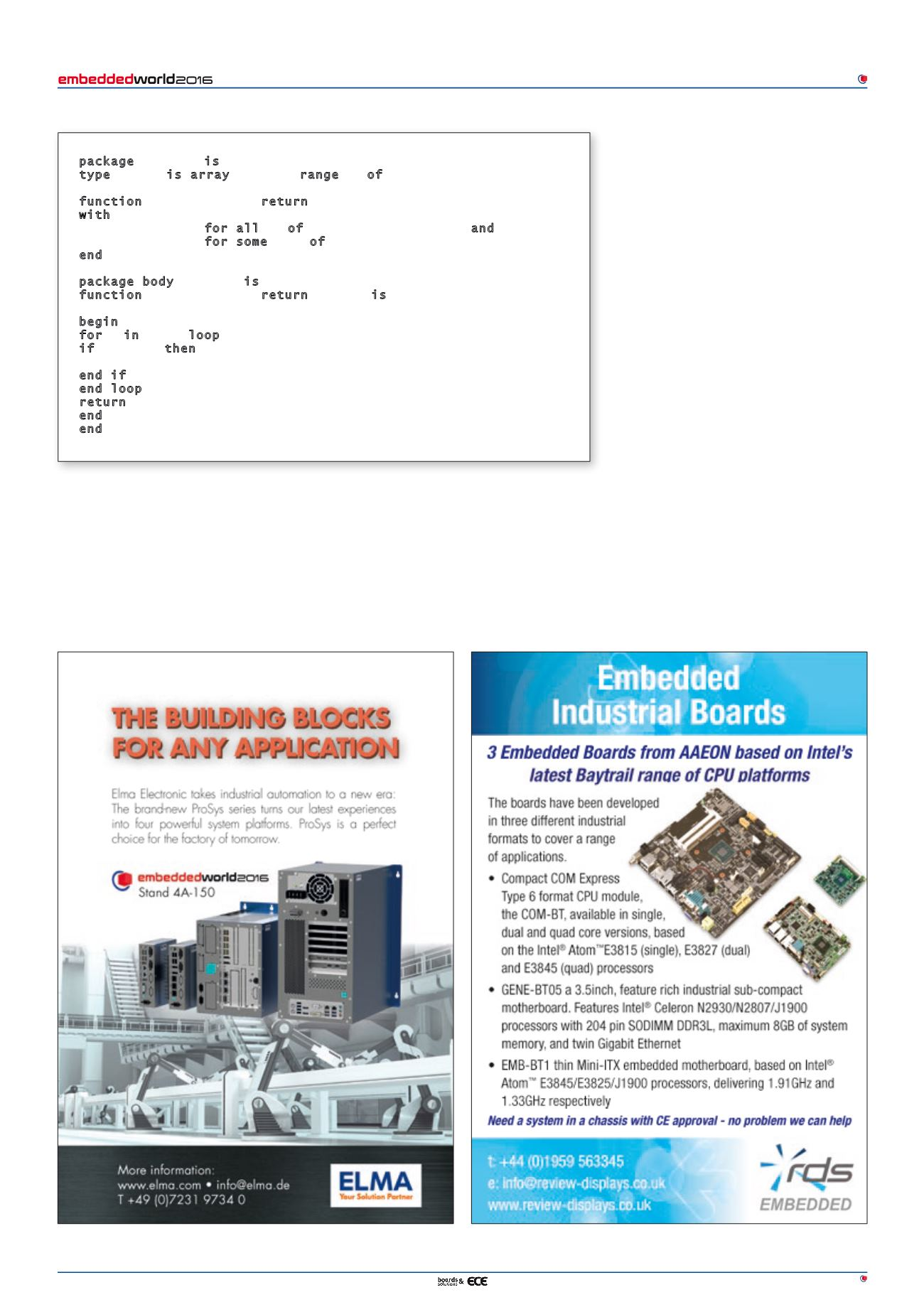# January/February 2016 - page 35February 2016
33
T
ools
& S
oftware
and ignored and regarded as a comment to
the human reader. And unlike the approach
found in other proof technologies, where the
contract notation is itself a separate specifica-
tion language, SPARK contracts use standard
Ada syntax and semantics. Proved statically
involves proving, at each point of call, that the
precondition is satisfied by the actual parame-
ter(s). It also means analyzing the subprogram
body and proving that, if the precondition is
satisfied and the subprogram terminates, then
the caller. If a contract cannot be proved, this
could mean either that the code is inconsistent
with the contract (so that one of them needs
to be corrected) or that there is not enough
information to prove that the contract will be
met. In the latter case the developer may be
able to supply additional information to assist
the proof engine.
Thanks to advances in proof technology and the
increased speed and capacity of modern com-
puters, formal methods are a practical static
analysis technique, and they do not require
expertise in mathematical logic. As illustration,
College in the US, with no previous experience
with Ada or formal methods, learned and used
SPARK to develop the software for a CubeSat
satellite that recently completed a successful
two-year orbital mission.
How might formal methods be applied in
practice, and in particular how can they be
combined with testing? Several scenarios are
possible; mixing and matching tested and
proved code is performed at subprogram call
boundaries.
In the case of testing only the contracts are
enabled for runtime checking during the tests
(Ada semantics); robustness tests can exer-
packageVector_Pkgis
type Vector is array(Positive range<>) of Integer;
function Min(V : Vector) return Integer
with Pre =>V'Length> 0,
Post => (for allItemof V =>Min'Result<= Item) and
(for some Item of V =>Min'Result = Item);
endVector_Pkg;
package bodyVector_Pkgis
function Min(V : Vector) return Integer is
M : Integer := Integer'Last;
-- Largest Integer value
begin
for I inV'Rangeloop
-- I ranges over the index values
if V(I) < M then
M := V(I);
end if;
end loop;
return M;
end Min;
endVector_Pkg;
Figure 2. Code example of a subprogram with precondition and postcondition contracts
1...,25,26,27,28,29,30,31,32,33,34 36,37,38,39,40,41,42,43,44,45,...58